Question

# Consider the reaction A ⟶ Products. The rate law for this reaction is rate = k[A]...

Consider the reaction A ⟶ Products. The rate law for this reaction is rate = k[A] where k= 3.00 X × 10-3 s-1 at a particular temperature. If the initial [A] = 0.500 M, what will be the concentration of A after 2.00 minutes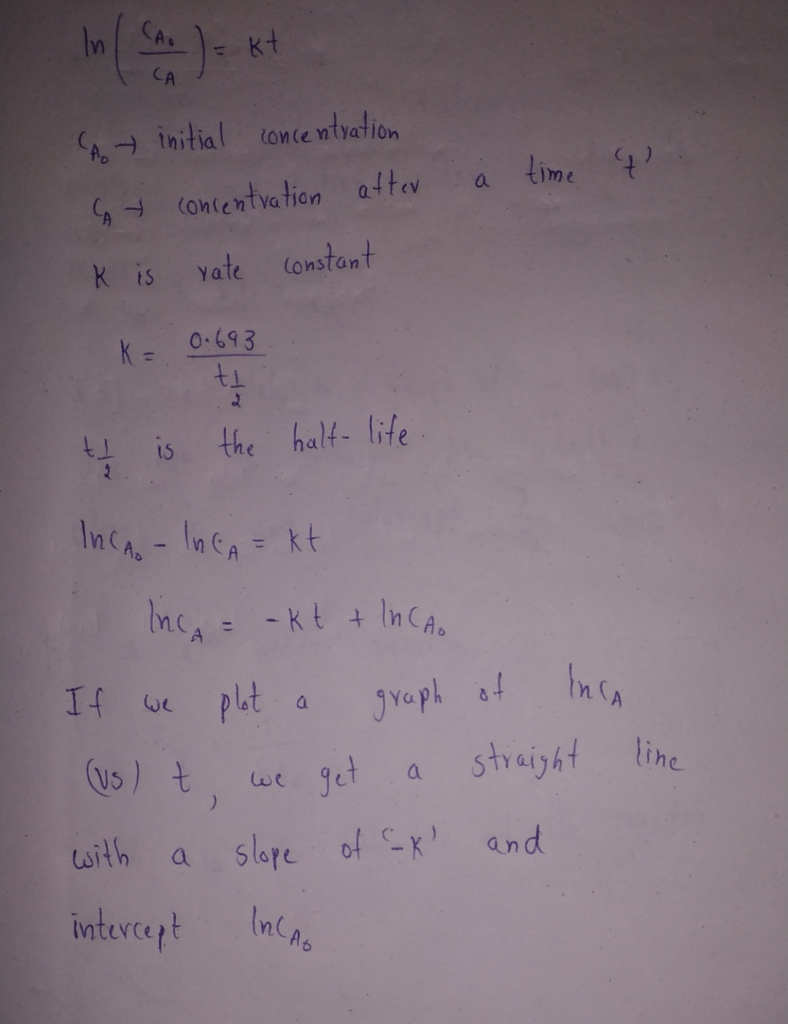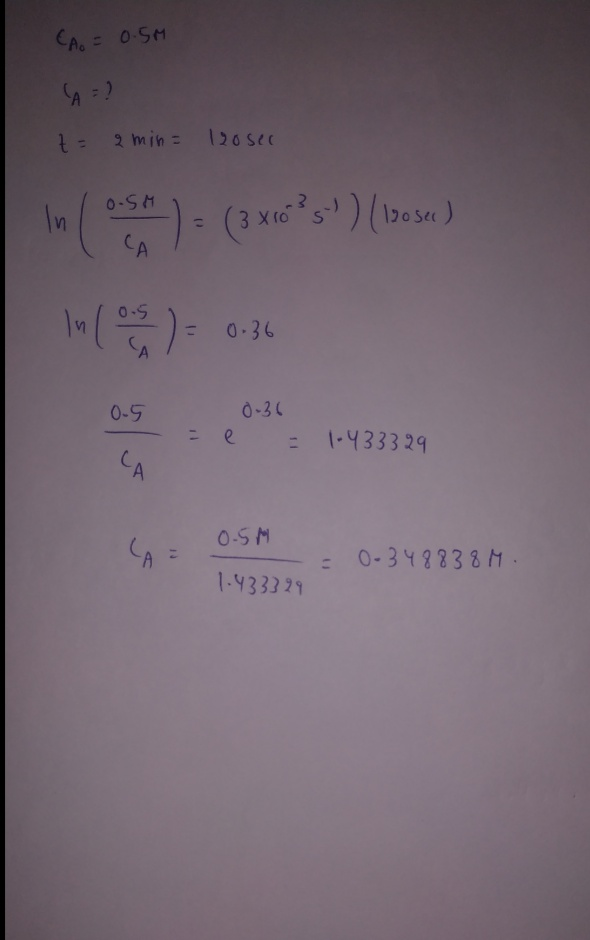#### Earn Coins

Coins can be redeemed for fabulous gifts.

Similar Homework Help Questions
• ### 4) A particular irreversible chemical reaction follows the rate law rate =k [A] At a particular...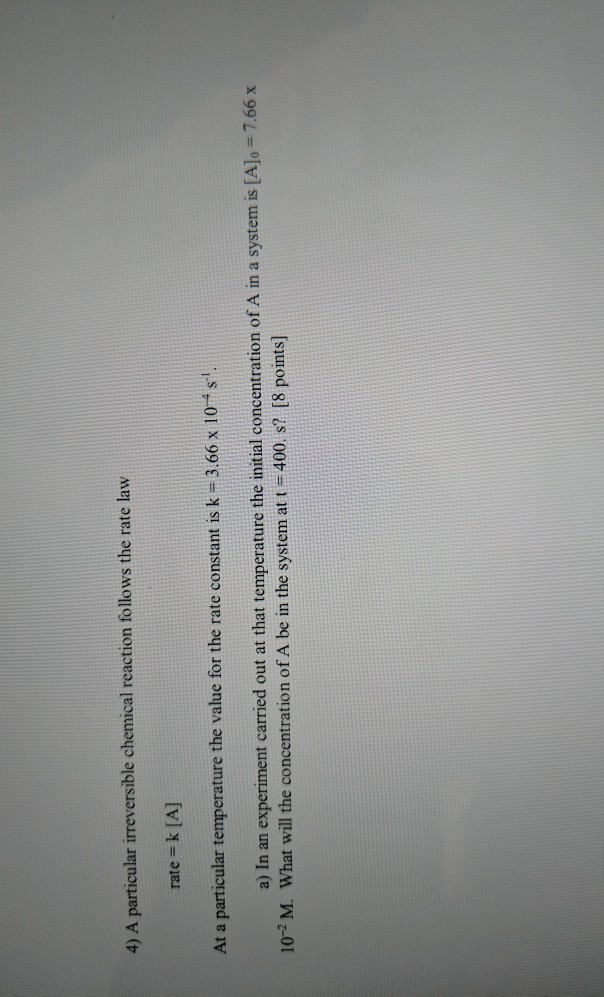4) A particular irreversible chemical reaction follows the rate law rate =k [A] At a particular temperature the value for the rate constant is k = 3.66 x 10-s. a) In an experiment carried out at that temperature the initial concentration of A in a system is [A]o = 7.66 x 10-2 M. What will the concentration of A be in the system at t = 400. s? [8 points]

• ### The rate law for the decomposition of N2O5 is rate = k[N2O5] If k = 1.0...

The rate law for the decomposition of N2O5 is rate = k[N2O5] If k = 1.0 x 10-5 s-1, what is the reaction rate when the N2O5 concentration is 0.0091 mol L-1? 2.The decomposition of acetaldehyde, CH3CHO, was determined to be a second order reaction with a rate constant of 0.0771 M-1 s-1. If the initial concentration of acetaldehyde is 0.301 M , what will the concentration be after selected reaction times? a. What will the CH3CHO concentration be after...

• ### A certain reaction A products has the rate law, rate = k . If the initila...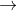A certain reaction A products has the rate law, rate = k . If the initila concentration of A is 4.00 M and the concentration of A after 2.00 min is 1.00 M, what is the rate constant?

• ### 4) A particular irreversible chemical reaction follows the rate law rate = k [A] At a...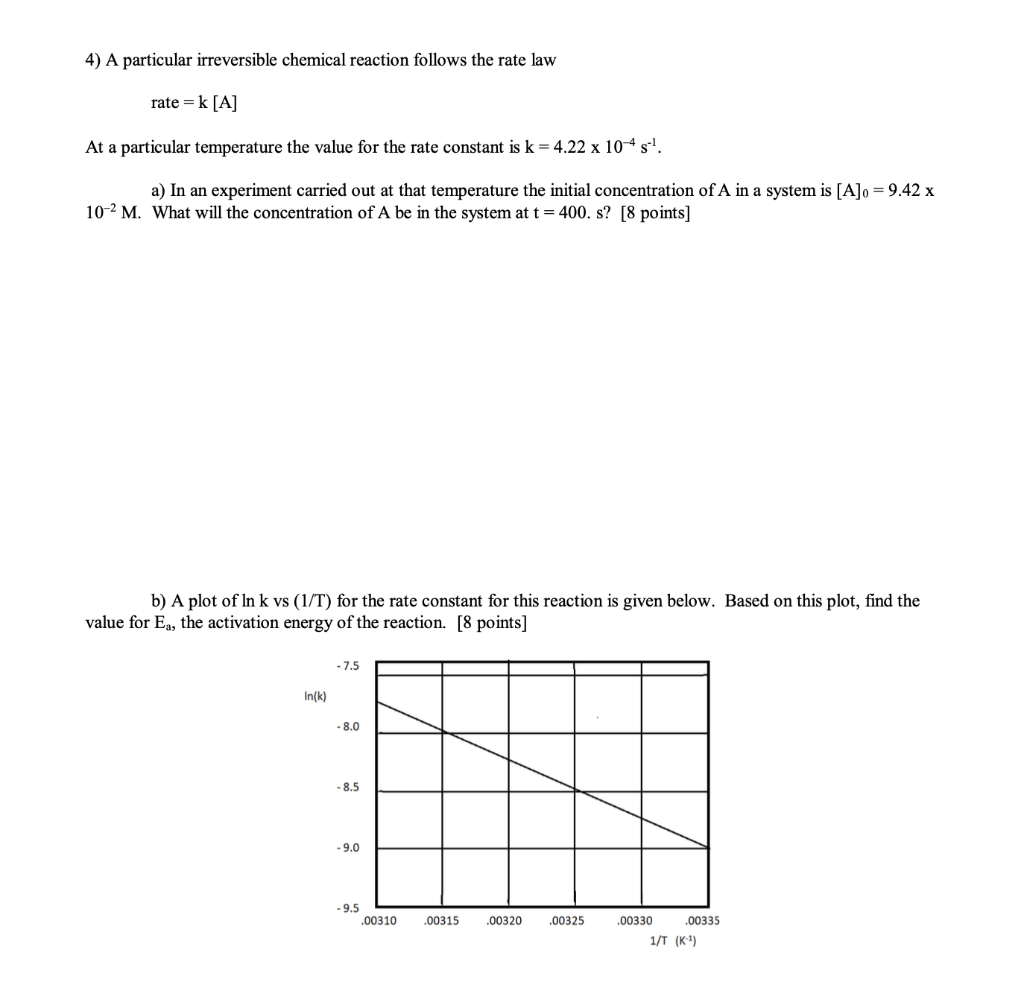4) A particular irreversible chemical reaction follows the rate law rate = k [A] At a particular temperature the value for the rate constant is k = 4.22 x 10-4s!. a) In an experiment carried out at that temperature the initial concentration of A in a system is [A]o = 9.42 x 10-2 M. What will the concentration of A be in the system at t = 400. s? [8 points) b) A plot of In k vs (1/T) for...

• ### 4) A particular irreversible chemical reaction follows the rate law rate = k[A] At a particular...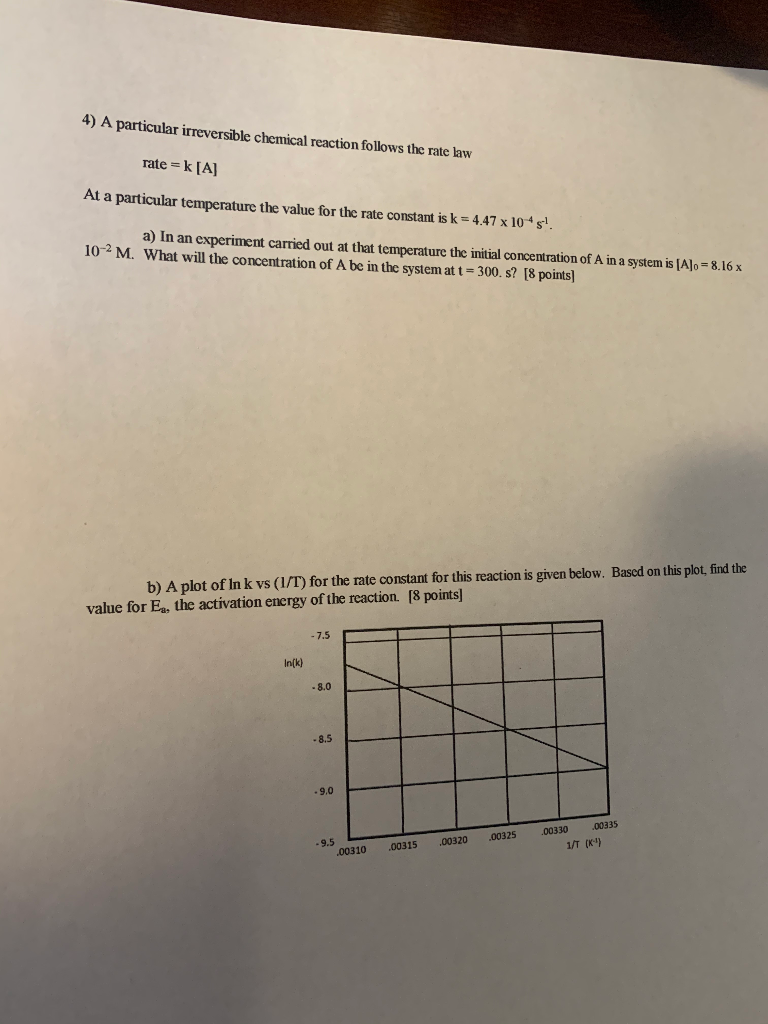4) A particular irreversible chemical reaction follows the rate law rate = k[A] At a particular temperature the value for the rate constant is k = 4.47 x 10*s! a) In an experiment carried out at that temperature the initial concentration of A in a system is [A]o = 8.16 x 10-2 M. What will the concentration of A be in the system at t = 300. s? [8 points) b) A plot of Ink vs (1/T) for the rate...

• ### urgent 4) A particular irreversible chemical reaction follows the rate law rate = k[A] At a...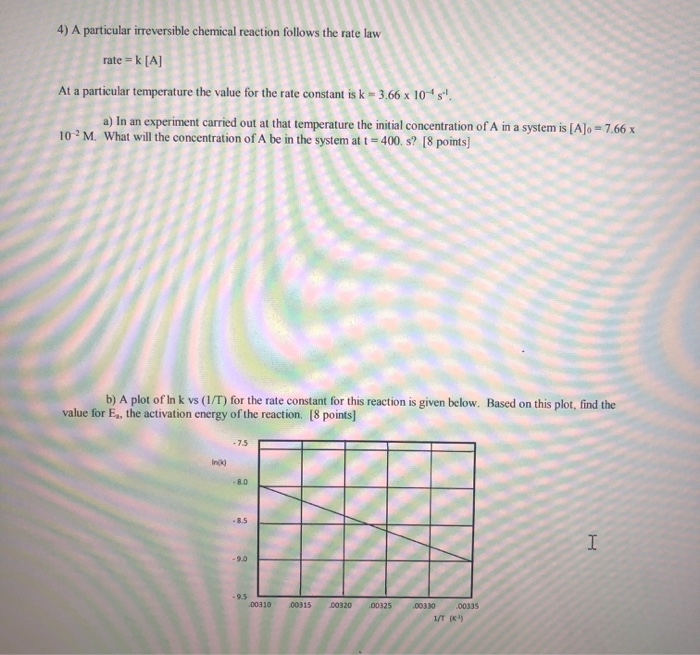urgent 4) A particular irreversible chemical reaction follows the rate law rate = k[A] At a particular temperature the value for the rate constant is k - 3.66 x 10-4s. a) In an experiment carried out at that temperature the initial concentration of A in a system is [A]o = 7.66 x 10 M. What will the concentration of A be in the system at t = 400.s? [8 points] b) A plot of Ink vs (1/T) for the rate...

• ### 4) A particular irreversible chemical reaction follows the rate law rate = k[A] At a particular...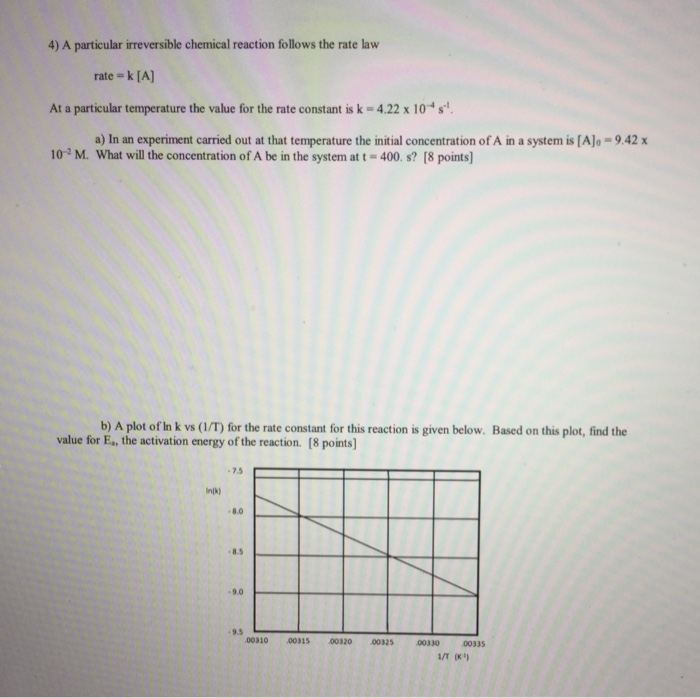4) A particular irreversible chemical reaction follows the rate law rate = k[A] At a particular temperature the value for the rate constant is k = 4.22 x 10+s! a) In an experiment carried out at that temperature the initial concentration of A in a system is [A]o = 9.42 x 102M. What will the concentration of A be in the system at t = 400.s? [8 points) b) A plot of Ink vs (1/T) for the rate constant for...

• ### For this question, consider the reaction A-->B with a rate constant k=7.17x10^-4 m/s. A.)In one experiment,...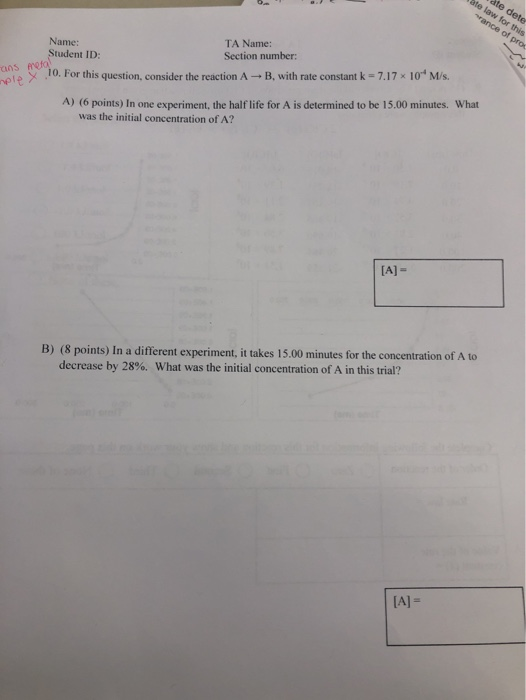For this question, consider the reaction A-->B with a rate constant k=7.17x10^-4 m/s. A.)In one experiment, the half life for A is determined to be 15.00 minutes. What was the initial concentration of A? B.) In a different experiment, it takes 15.00 minutes for the concentration of A to decrease by 28%. What was the initial concentration of A in this trial? ate dete ate law for this rance of pros Name: TA Name: Student ID: Section number: Aneta 10For...

• ### Consider the reaction A + B ? products From the following data obtained at a certain...

Consider the reaction A + B ? products From the following data obtained at a certain temperature, determine the rate law, the order of the reaction, and calculate the rate constant k. Experiment 1: [A] = 1.50 M; [B] = 1.50 M; Initial Rate = 3.20 x 10-1 M/s Experiment 2: [A] = 1.50 M; [B] = 2.50 M; Initial Rate = 3.20 x 10-1 M/s Experiment 3: [A] = 3.00 M; [B] = 1.50 M; Initial Rate = 6.40...

• ### The rate law for the reaction 3A(g) → C(g) is rate-kAI2 where k = 4.36 x...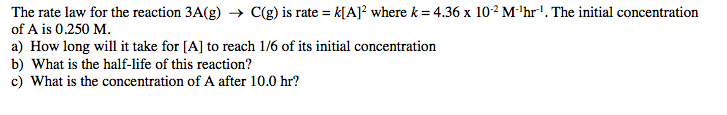The rate law for the reaction 3A(g) → C(g) is rate-kAI2 where k = 4.36 x 10-2 Mhrl. The initial concentration of A is 0.250 M. a) How long will it take for [A] to reach 1/6 of its initial concentration b) What is the half-life of this reaction? c) What is the concentration of A after 10.0 hr?